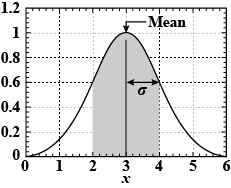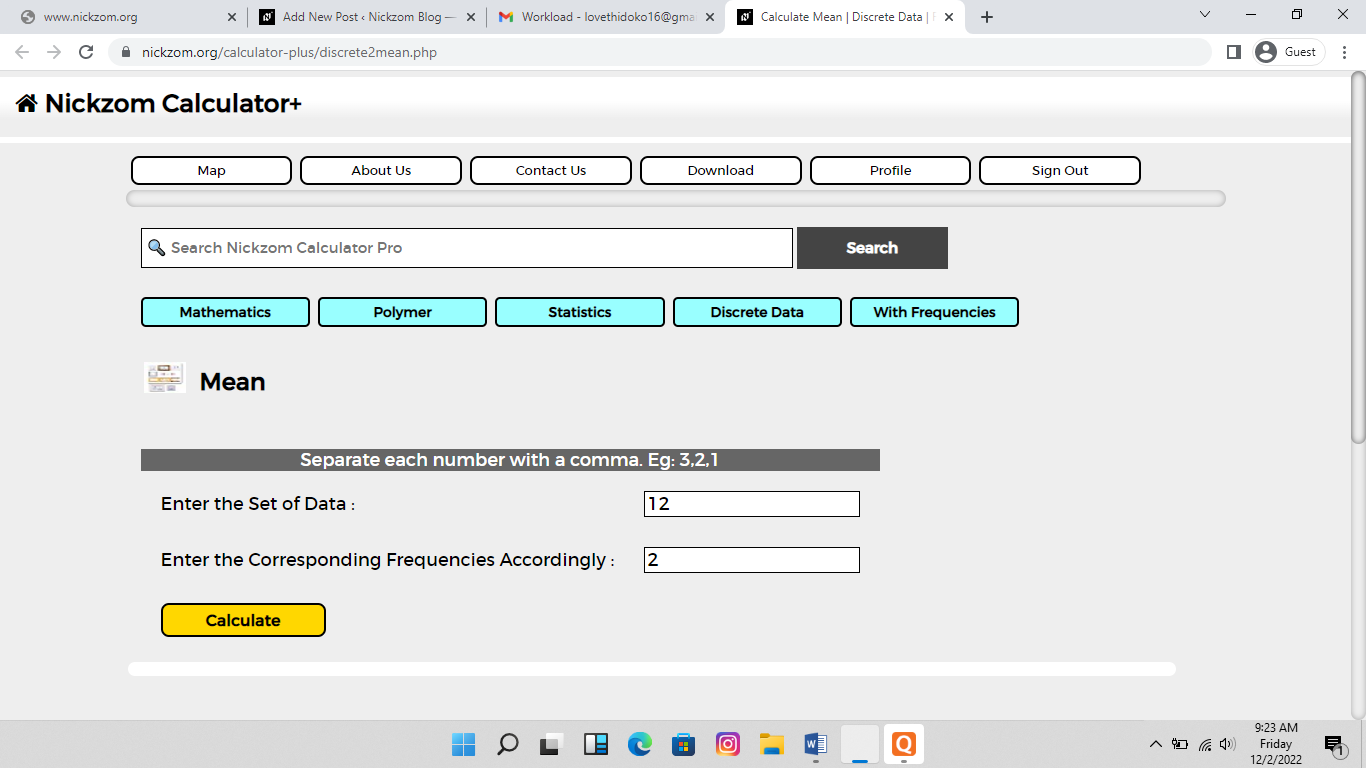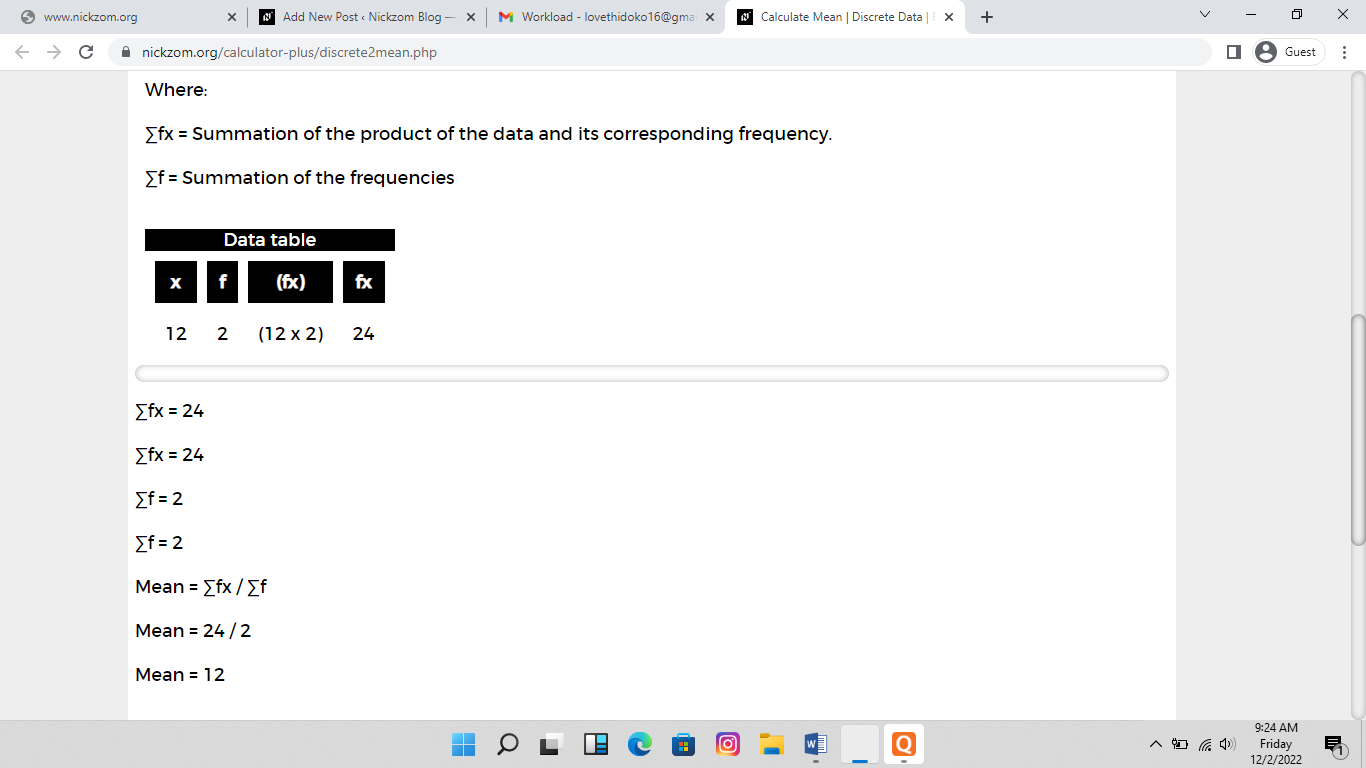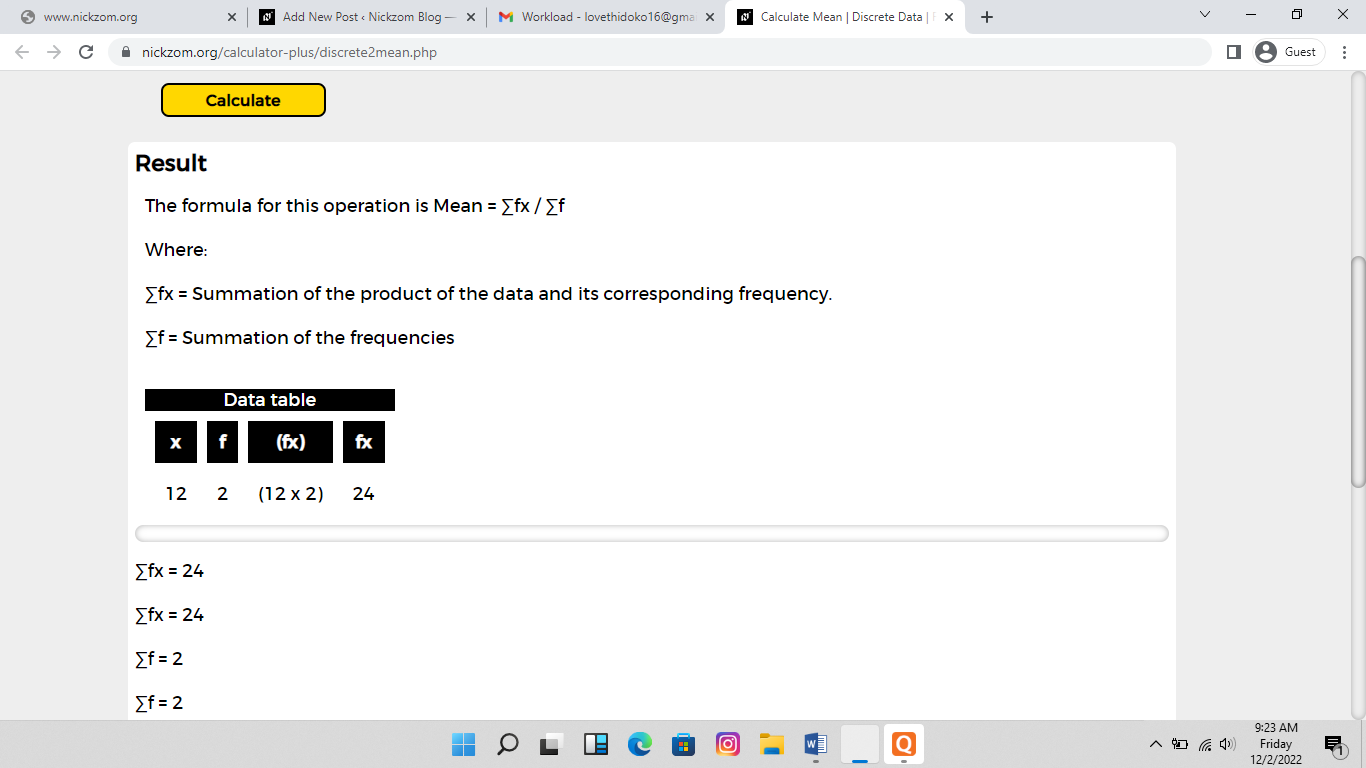# How to Calculate and Solve for Number Average Molecular Weight | Polymer

The image of mean is represented belowTo compute for mean, two essential parameters are needed and these parameters are Set of data and Corresponding frequencies accordingly.

The formula for calculating mean:

Mean = ∑fx / ∑f

Where;

∑fx = Summation of the product of the data and its corresponding frequency.
∑f = Summation of the frequencies

Let’s solve an example;
Find the mean when the set of data is 12 and the corresponding frequencies accordingly is 2.

This implies that;

x f (fx) fx
12 2 (12 x 2) 24

∑fx = 24
∑f = 2

Mean = ∑fx / ∑f
Mean = 24 / 2
Mean = 12

Therefore, the mean is 12.

Nickzom Calculator – The Calculator Encyclopedia is capable of calculating the mean.

To get the answer and workings of the mean using the Nickzom Calculator – The Calculator Encyclopedia. First, you need to obtain the app.

You can get this app via any of these means:

You can also try the demo version via https://www.nickzom.org/calculator

Apple (Paid) – https://itunes.apple.com/us/app/nickzom-calculator/id1331162702?mt=8
Once, you have obtained the calculator encyclopedia app, proceed to the Calculator Map, then click on Materials and Metallurgical under Engineering.Now, Click on Polymer under Materials and MetallurgicalNow, Click on Number Average Molecular Weight under PolymerThe screenshot below displays the page or activity to enter your values, to get the answer for the number average molecular weight according to the respective parameter which is the Set of data and Corresponding frequencies accordingly.Now, enter the values appropriately and accordingly for the parameters as required by the Set of data is 12 and Corresponding frequencies accordingly is 2.Finally, Click on CalculateAs you can see from the screenshot above, Nickzom Calculator– The Calculator Encyclopedia solves for the number average molecular weight and presents the formula, workings and steps too.